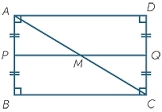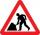# Area of a rectangle

Calculate a rectangle area with a diagonal of u = 12.5cm and a width of b = 3.5cm. Use the Pythagorean theorem.

S =  42 cm2

### Step-by-step explanation:Did you find an error or inaccuracy? Feel free to write us. Thank you!Tips to related online calculators
Pythagorean theorem is the base for the right triangle calculator.

#### You need to know the following knowledge to solve this word math problem:

We encourage you to watch this tutorial video on this math problem:

## Related math problems and questions:

• RectangleThere is a rectangle with a length of 12 cm and a diagonal 8 cm longer than the width. Calculate the area of a rectangle.
• Diagonal - simpleCalculate the length of the diagonal of a rectangle with dimensions 5 cm and 12 cm.
• Rectangle 3-4-5The sides of the rectangle are in a ratio of 3:4. The length of the rectangle diagonal is 20 cm. Calculate the content of the rectangle.
• Prism - boxThe base of prism is a rectangle with a side of 7.5 cm and 12.5 cm diagonal. The volume of the prism is V = 0.9 dm3. Calculate the surface of the prism.
• The sidesThe sides of the rectangle are in a ratio of 3: 5 and its circumference measures 72 cm. Calculate: a) the size of both sides of the rectangle b) the area of the rectangle c) the length of the diagonals
• Rectangle diagonalThe rectangle, one side of which is 5 cm long, is divided by a 13 cm diagonal into two triangles. Calculate the area of one of these triangles in cm2.
• Angle of diagonalsCalculate the perimeter and the area of a rectangle if its diagonal is 14 cm and the diagonals form an angle of 130°.
• RectangleThe length of the rectangle are in the ratio 5:12 and the circumference is 238 cm. Calculate the length of the diagonal and area of rectangle.
• Isosceles trapezoidThe lengths of the bases of the isosceles trapezoid are in the ratio 5:3, the arms have a length of 5 cm and height = 4.8 cm. Calculate the circumference and area of a trapezoid.
• Diagonal to areaCalculate the area of a rectangle in which the length of the diagonal is 10 cm.
• Rectangle 35Find the area of a rectangle when the diagonal is equal to 30 cms and the width is double the length.
• Side and diagonalFind the circumference and the area of the rectangle if given: side a = 8 cm diagonal u = 10 cm.
• BillboardRectangular billboard is 2.5 m long with a diagonal 2.8 m long. Calculate the perimeter and the content area of the billboard.
• Rectangular trapezoidCalculate the content of a rectangular trapezoid with a right angle at the point A and if |AC| = 4 cm, |BC| = 3 cm and the diagonal AC is perpendicular to the side BC.
• RhombusCalculate the perimeter and area of ​​a rhombus whose diagonals are 39 cm and 51 cm long.
• DiagonalCalculate the diagonal length of the rectangle ABCD with sides a = 8 cm, b = 7 cm.
• Isosceles triangleCalculate area and perimeter of an isosceles triangle ABC with base AB if a = 6 cm, c = 7 cm.